目光所及，心之所悦！作为宝马品牌旗下的中流砥柱车型，BMW 5系在过去的45年间成功赢取了超过800万客户的青睐。今年上市的第7代全新BMW 5系以更加优雅动感的姿态赢得了全球瞩目。作为汽车设计潮流的引领者，BMW 5系在不同年代都专注而执着地做着同一件事：用前瞻的设计理念诠释对“豪华”、“商务”、“运动”、“优雅”的理解。全新一代BMW 5系的横空出世引来举世瞩目，其设计之美不仅止于陶醉你的感官，它工程师灵魂赋予的功能之美同样值得喝彩！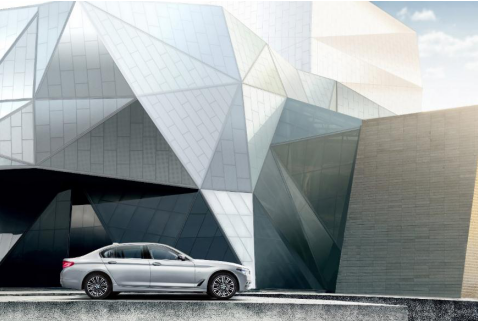全新BMW 5系Li拥有完美的车身比例与精致的车头设计，在车流中永远都是瞩目的焦点。作为车头最为夺目的亮点，全新设计的全LED大灯在宝马家族设计基因的基础上融入了新的元素，整体造型变为了非封闭式六边形天使眼，为整体车头设计画上了点睛之笔，而大灯内侧的小型弯角细节处理体现出宝马设计师对质感的不懈追求。
此外，标志性双肾格栅作为BMW最具辨识度的家族设计符号之一，被全新BMW 5系Li重新勾勒。通过对双肾轮廓、镀铬边框以及双肾内部竖直饰条的优化，车头看起来更加立体精致。优雅造型背后，第三代降组进气格栅中的气孔会根据发动机、刹车等部件的工况所需的空气量进行自动开合调节，最大限度的确保强劲动力的输出并降低空气阻力和燃油消耗。一开一合间不仅体现了设计与功能的完美结合，更让驾驶者始终感受到全新BMW 5系Li强劲动力带来的驾驶愉悦。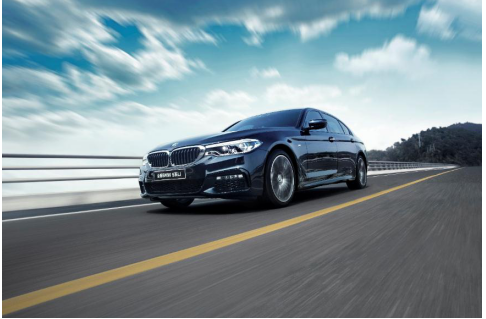同时，位于前轮前部的空气气帘设计配合前轮后部的鲨鱼鳃状导气装置，不仅增加了细节设计的质感，更为优异的空气动力学表现做出了巨大贡献。空气气帘采用逐渐收紧的结构设计，这种方式一方面可以有效降低空气阻力从而达到更低油耗、更低二氧化碳排放的目的；另一方面还能加速空气更快的通过车轮，为刹车系统进行有效的降温。精巧的设计确保刹车系统始终处于亢奋状态，让驾驶者充分享受激情驾驭的快感。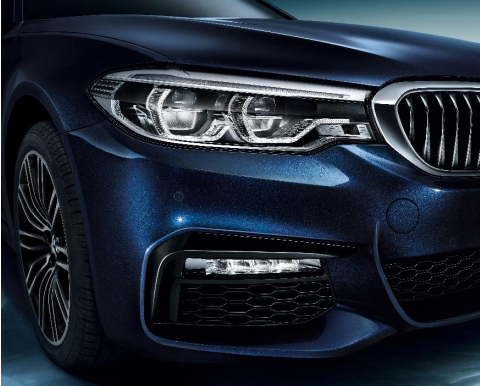从车身造型呈现出的整体效果看，两条相互呼应的双腰线设计与霍夫麦斯特弯角融为一体，形成了全新一代5系侧面最突出的车身设计特征。与上一代车型贯穿车身侧面的单腰线相比，全新BMW 5系Li用一条始于前轮眉顶部上扬的腰部线条突出了车身侧面的运动气质，另外一条近乎水平的腰线贯穿车身中部，增加了车身的视觉稳定感。两条上下呼应的腰线诠释了全新BMW 5系Li的设计主旨，即寻求优雅与运动的平衡。另外，双腰线设计对制造工艺提出了更高的要求，也让全新BMW 5系Li的车身侧面呈现出了更为立体的视觉效果，特别是在恰当的光线下，车身反射出的光与影的质感即是汽车设计领域的至高境界：让光影升华设计线条的至美追求。此外，由鲨鱼鳃延伸出的线条贯穿前后车门底部，体现出了全新BMW 5系区别于7系的独特气质。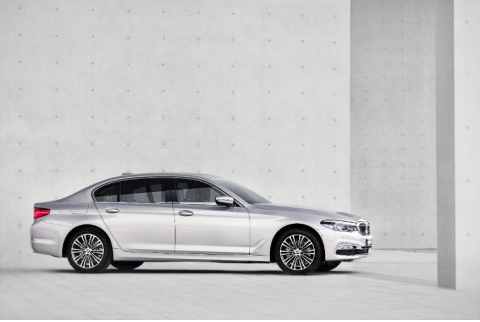进到车内，全新BMW 5系Li的内饰体现了BMW一贯的豪华优雅并充满格调的气质。作为重视驾驶者使用感受的BMW品牌，全新BMW 5系Li的驾驶舱设计延续了偏向驾驶员一侧的中控台设计理念，所有功能按键的设计都触手可及，操作时极大地减少了视线偏移量，有效地提升了驾乘人员的安全。全新一代全彩平视系统的加入更是将驾驶者视线进一步停留在道路前方，其投射面积较上一代增大70%，最大限度的降低由于驾驶者注意力偏移发生危险的概率。“设计服务于功能”的BMW设计理念，在这一点上同样体现地淋漓尽致。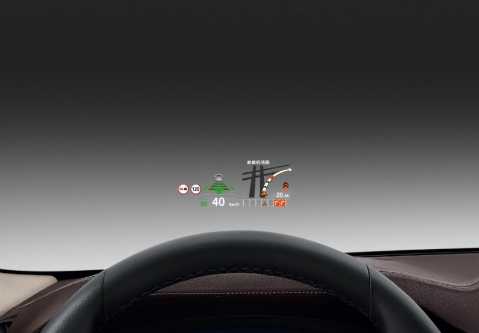除此之外，全新BMW 5系Li内饰设计的科技氛围再次升级，这与日趋年轻化的精英用户群体特征非常契合。中控台上部的10.25寸悬浮屏幕取代了老款嵌入式屏幕，而在显示技术方面同样采用宝马最新的ID6系统，其界面风格一改之前的竖屏列表排列方式，横屏图标显示不仅可视性更强，同样增加了视觉科技感。12.3寸的可编程全液晶仪表盘也是目前主流豪华品牌中的上乘之作。同时，用户体验一致受到好评的最新iDrive智能人机交互系统支持自然语音识别、手势操作等5种交互方式，让日常使用更加便捷，充分展现了科技改变生活的创新魅力。此外，全新的方向盘细节表现再配合中控台不同材质饰板的精心搭配，无不展现着BMW设计师对豪华品质的独到见解。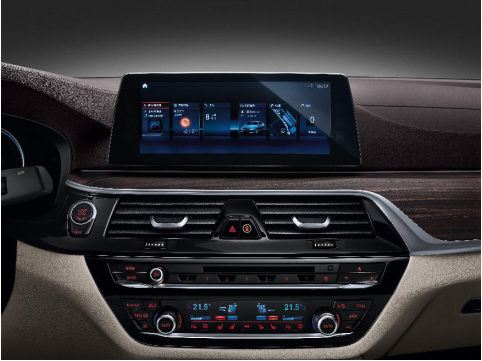在烘托车内氛围方面，全新BMW 5系Li充分体现了当下时代新精英阶层对科技感的畅想。拉开车门即璀璨流泻的迎宾光毯令尊贵感油然而生，环绕内饰四周的11种可调色LED氛围灯让夜晚的驾驶充满了情调，香氛系统的加入更恰到好处的让人沉醉其中流连忘返，时刻处于舒适惬意的乘坐状态。更值得一提的是，中国市场独有的星空全景天窗在同级车型中也是独树一帜，堪比超豪华“双R”品牌车型的视觉效果增添了车内无限的豪华浪漫之光。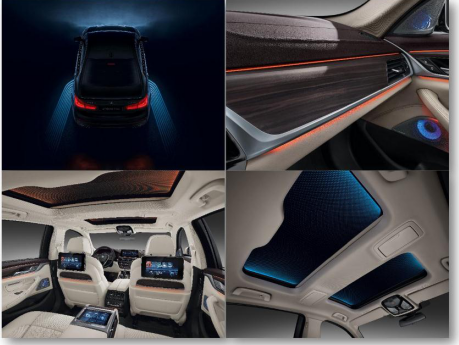设计之美，见仁见智。超过800万拥趸者背后一定有BMW 5系独到之处，需细细品味。作为全新一代车型的入门款，BMW 528Li上市特别款以44.99万的亲民价格进一步拉近了与精英群体的距离。在下一个拐角，你可能就会遇到全新BMW 5系Li，看它的设计之美如何有力地绽放！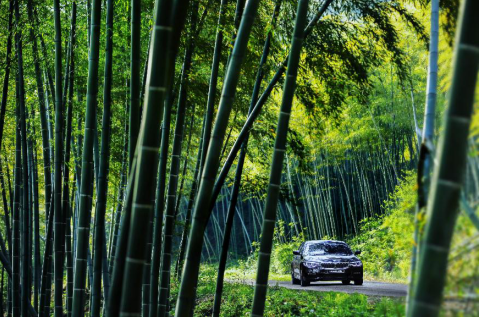【关于北京宝诚】
北京宝诚百旺汽车销售服务有限公司是华晨宝马与德国宝马共同授权的BMW特许经销商。北京宝诚自成立以来，一直将客户满意度放在第一位，是集新车销售、售后服务、零配件供应、金融贷款、保险服务、大客户业务及二手车置换为一体的BMW专业销售服务中心。

##### 相关资讯

|分享

•已阅！握爪
•我手滑为你点赞
•128个赞！
•不明觉厉
•阅后既醉
•有钱！任性
•照片太美，我不敢看
•干货！杠杠的
•高大上
•膜拜中！
•请接受我的膝盖
•猫哥/猫妹么么哒
•神吐槽
•我只笑笑不说话
•我想静静
•窒息
##### 资讯相关车系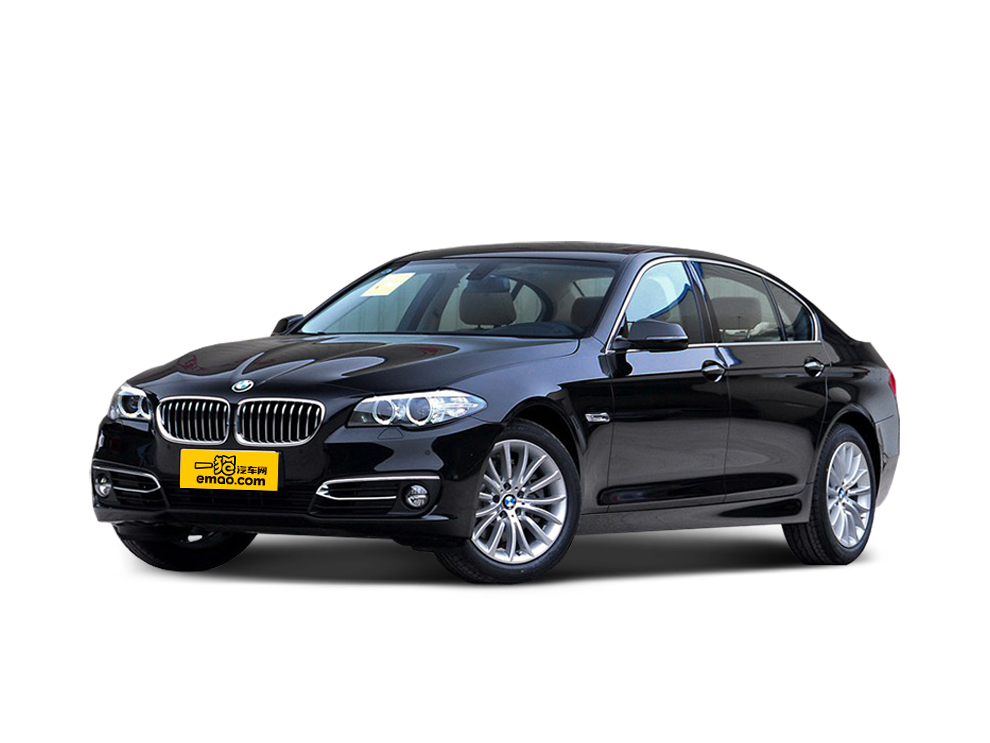##### 热度排行• 阿斯顿·马丁
• 奥迪
• 阿尔法罗密欧
• ALPINA

• 巴博斯
• 宝骏
• 宝马
• 保时捷
• 北汽制造
• 奔驰
• 奔腾
• 本田
• 比亚迪
• 标致
• 别克
• 宾利
• 布加迪
• 北汽威旺
• 北京
• 北汽绅宝
• 北汽幻速
• 北汽新能源
• 宝沃
• 比速汽车
• 北汽道达

• 昌河
• 长安
• 长城
• 长安商用
• 成功汽车
• 长江EV
• 长安轻车型

• 大众
• 道奇
• 东风
• 东风风神
• 东风小康
• 东南
• DS
• 东风风行
• 东风风度
• 东风风光

• 法拉利
• 菲亚特
• 丰田
• 福迪
• 福特
• 福田汽车
• 福汽启腾
• 风诺

• GMC
• 广汽传祺
• 广汽吉奥
• 观致

• 哈飞
• 海格
• 海马
• 华泰
• 黄海
• 恒天
• 红旗
• 哈弗
• 华颂
• 华凯
• 华泰新能源
• 汉腾汽车

• Jeep
• 江淮
• 江铃
• 捷豹
• 金杯
• 九龙
• 吉利汽车
• 金旅
• 金龙
• 江铃集团轻汽
• 江铃集团新能源
• 君马汽车
• 捷途

• 开瑞
• 凯迪拉克
• 科尼赛克
• 克莱斯勒
• KTM
• 卡威
• 凯翼
• 康迪
• 康迪电动汽车集团

• 猎豹汽车
• 兰博基尼
• 劳斯莱斯
• 雷克萨斯
• 雷诺
• 理念
• 力帆
• 莲花汽车
• 林肯
• 铃木
• 陆风
• 路虎
• 路特斯
• 领志
• 领克
• 零跑汽车

• MG
• MINI
• 马自达
• 玛莎拉蒂
• 迈凯伦
• 摩根

• 纳智捷

• 讴歌
• 欧宝
• 欧朗
• 欧拉
• 欧尚汽车

• 帕加尼

• 奇瑞
• 启辰
• 起亚
• 前途
• 庆铃汽车

• 日产
• 荣威
• 瑞麒汽车
• 如虎
• 瑞驰

• 上汽大通
• smart
• 三菱
• 双环
• 双龙
• 斯巴鲁
• 斯柯达
• 萨博
• 思铭
• 赛麟
• SWM斯威汽车

• TESLA
• 泰卡特
• 腾势

• 威麟
• 威兹曼
• 沃尔沃
• 五菱汽车
• 五十铃
• 潍柴英致
• WEY
• 蔚来
• 威马汽车

• 现代
• 雪佛兰
• 雪铁龙
• 西雅特
• 新特汽车
• 小鹏汽车

• 野马汽车
• 一汽
• 依维柯
• 英菲尼迪
• 永源
• 驭胜

• 中华
• 中兴
• 众泰
• 知豆
• 之诺
• 正道汽车
• A
• B
• C
• D
• E
• F
• G
• H
• I
• J
• K
• L
• M
• N
• O
• P
• Q
• R
• S
• T
• U
• V
• W
• X
• Y
• Z

• 阿斯顿·马丁
• 奥迪
• 阿尔法罗密欧
• ALPINA

• 巴博斯
• 宝骏
• 宝马
• 保时捷
• 北汽制造
• 奔驰
• 奔腾
• 本田
• 比亚迪
• 标致
• 别克
• 宾利
• 布加迪
• 北汽威旺
• 北京
• 北汽绅宝
• 北汽幻速
• 北汽新能源
• 宝沃
• 比速汽车
• 北汽道达

• 昌河
• 长安
• 长城
• 长安商用
• 成功汽车
• 长江EV
• 长安轻车型

• 大众
• 道奇
• 东风
• 东风风神
• 东风小康
• 东南
• DS
• 东风风行
• 东风风度
• 东风风光

• 法拉利
• 菲亚特
• 丰田
• 福迪
• 福特
• 福田汽车
• 福汽启腾
• 风诺

• GMC
• 广汽传祺
• 广汽吉奥
• 观致

• 哈飞
• 海格
• 海马
• 华泰
• 黄海
• 恒天
• 红旗
• 哈弗
• 华颂
• 华凯
• 华泰新能源
• 汉腾汽车

• Jeep
• 江淮
• 江铃
• 捷豹
• 金杯
• 九龙
• 吉利汽车
• 金旅
• 金龙
• 江铃集团轻汽
• 江铃集团新能源
• 君马汽车
• 捷途

• 开瑞
• 凯迪拉克
• 科尼赛克
• 克莱斯勒
• KTM
• 卡威
• 凯翼
• 康迪
• 康迪电动汽车集团

• 猎豹汽车
• 兰博基尼
• 劳斯莱斯
• 雷克萨斯
• 雷诺
• 理念
• 力帆
• 莲花汽车
• 林肯
• 铃木
• 陆风
• 路虎
• 路特斯
• 领志
• 领克
• 零跑汽车

• MG
• MINI
• 马自达
• 玛莎拉蒂
• 迈凯伦
• 摩根

• 纳智捷

• 讴歌
• 欧宝
• 欧朗
• 欧拉
• 欧尚汽车

• 帕加尼

• 奇瑞
• 启辰
• 起亚
• 前途
• 庆铃汽车

• 日产
• 荣威
• 瑞麒汽车
• 如虎
• 瑞驰

• 上汽大通
• smart
• 三菱
• 双环
• 双龙
• 斯巴鲁
• 斯柯达
• 萨博
• 思铭
• 赛麟
• SWM斯威汽车

• TESLA
• 泰卡特
• 腾势

• 威麟
• 威兹曼
• 沃尔沃
• 五菱汽车
• 五十铃
• 潍柴英致
• WEY
• 蔚来
• 威马汽车

• 现代
• 雪佛兰
• 雪铁龙
• 西雅特
• 新特汽车
• 小鹏汽车

• 野马汽车
• 一汽
• 依维柯
• 英菲尼迪
• 永源
• 驭胜

• 中华
• 中兴
• 众泰
• 知豆
• 之诺
• 正道汽车
• A
• B
• C
• D
• E
• F
• G
• H
• I
• J
• K
• L
• M
• N
• O
• P
• Q
• R
• S
• T
• U
• V
• W
• X
• Y
• Z

• 阿斯顿·马丁
• 奥迪
• 阿尔法罗密欧
• ALPINA

• 巴博斯
• 宝骏
• 宝马
• 保时捷
• 北汽制造
• 奔驰
• 奔腾
• 本田
• 比亚迪
• 标致
• 别克
• 宾利
• 布加迪
• 北汽威旺
• 北京
• 北汽绅宝
• 北汽幻速
• 北汽新能源
• 宝沃
• 比速汽车
• 北汽道达

• 昌河
• 长安
• 长城
• 长安商用
• 成功汽车
• 长江EV
• 长安轻车型

• 大众
• 道奇
• 东风
• 东风风神
• 东风小康
• 东南
• DS
• 东风风行
• 东风风度
• 东风风光

• 法拉利
• 菲亚特
• 丰田
• 福迪
• 福特
• 福田汽车
• 福汽启腾
• 风诺

• GMC
• 广汽传祺
• 广汽吉奥
• 观致

• 哈飞
• 海格
• 海马
• 华泰
• 黄海
• 恒天
• 红旗
• 哈弗
• 华颂
• 华凯
• 华泰新能源
• 汉腾汽车

• Jeep
• 江淮
• 江铃
• 捷豹
• 金杯
• 九龙
• 吉利汽车
• 金旅
• 金龙
• 江铃集团轻汽
• 江铃集团新能源
• 君马汽车
• 捷途

• 开瑞
• 凯迪拉克
• 科尼赛克
• 克莱斯勒
• KTM
• 卡威
• 凯翼
• 康迪
• 康迪电动汽车集团

• 猎豹汽车
• 兰博基尼
• 劳斯莱斯
• 雷克萨斯
• 雷诺
• 理念
• 力帆
• 莲花汽车
• 林肯
• 铃木
• 陆风
• 路虎
• 路特斯
• 领志
• 领克
• 零跑汽车

• MG
• MINI
• 马自达
• 玛莎拉蒂
• 迈凯伦
• 摩根

• 纳智捷

• 讴歌
• 欧宝
• 欧朗
• 欧拉
• 欧尚汽车

• 帕加尼

• 奇瑞
• 启辰
• 起亚
• 前途
• 庆铃汽车

• 日产
• 荣威
• 瑞麒汽车
• 如虎
• 瑞驰

• 上汽大通
• smart
• 三菱
• 双环
• 双龙
• 斯巴鲁
• 斯柯达
• 萨博
• 思铭
• 赛麟
• SWM斯威汽车

• TESLA
• 泰卡特
• 腾势

• 威麟
• 威兹曼
• 沃尔沃
• 五菱汽车
• 五十铃
• 潍柴英致
• WEY
• 蔚来
• 威马汽车

• 现代
• 雪佛兰
• 雪铁龙
• 西雅特
• 新特汽车
• 小鹏汽车

• 野马汽车
• 一汽
• 依维柯
• 英菲尼迪
• 永源
• 驭胜

• 中华
• 中兴
• 众泰
• 知豆
• 之诺
• 正道汽车
• A
• B
• C
• D
• E
• F
• G
• H
• I
• J
• K
• L
• M
• N
• O
• P
• Q
• R
• S
• T
• U
• V
• W
• X
• Y
• Z